Applied and Computational Mathematics
Volume 4, Issue 3, June 2015, Pages: 174-180

Option Pricing Variance Reduction Techniques Under the Levy Process

Li Zhou1, Hong Zhang1, Jian Guo1, Shucong Ming2

1School of Information, Beijing Wuzi University, Beijing, China

2Chinese Academy of Finance and Development, Central University of Finance and Economics, Beijing, China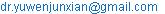(Hong Zhang)

Li Zhou, Hong Zhang, Jian Guo, Shucong Ming. Option Pricing Variance Reduction Techniques Under the Levy Process. Applied and Computational Mathematics. Vol. 4, No. 3, 2015, pp. 174-180. doi: 10.11648/j.acm.20150403.20

Abstract: After the 2008 financial crisis, the global derivatives trading volume in options proportion is growing, more and more investors build portfolios using options to hedge or arbitrage, our futures and stock options will soon open. Theoretical research of options is also changing, option pricing models under Levy processes developed rapidly. In this context, a review of the China's warrants market and the introduction of option pricing models can not only help us to reflect Chinese financial derivatives market regulation, but also to explore the option pricing theory for China`s financial market environment. In the framework of Monte Carlo simulation pricing, we established mufti-Levy process option pricing models, the structural model for the given parameter estimation and risk-neutral adjustment method are discussed, the last part of this chapter is an empirical analysis of China warrants trading data in order to prove the validate of Levy models. Key word: Levy stochastic processes, option pricing models, Chinese warrants market, American option pricing, risk-neutral adjustment, variance reduction techniques.

Keywords: Option Pricing, Variance Reduction Techniques, Levy Process

1. Introduction

The theoretical basis of Monte Carlo simulation is on the Law of Large Number. This law guarantees we can estimate the real value by the sample mean after enough times’ random simulation. And the errors of estimation can gradually converge with the increase in simulation times. But the convergence rate is negatively correlated with the sample variance, which implies that estimates by Monte Carlo simulation can be more accurate if the variances between the simulation values, with the simulation times being equal. Thus, the reduction of samples’ variance is key to improving the estimation’s precision. The incumbent techniques to reduce variances include Antithetic Variables Method, Importance Sampling, Control Variates Method, etc. Note that the core of the Antithetic Variables Method is to take the random numbers pair by pair and to ensure that each pair of random numbers is negatively associated with the mean. This method appears to be easy to operate. Importance Sampling Method endows each sample point with a weight to reduce the variances by transforming the measures. Control Variables Method helps to reduce the variance by selecting and artificially constructing an instrumental variable through the correlations of instrumental variables and original variables.

This chapter is focusing on the generating algorithm of time-varying Brown Motion in Levy Process and introducing the Control Variables Method to deal with the reduction of sample points’ variances of Levy random numbers which to a great extent improves the efficiency of Levy option pricing model. Furthermore, owing to the fact that the algorithm should be matched up with multi-dimensional random numbers, this algorithm also considers the quasi Monte Carlo simulation technique, which generates the multi-dimensional variables in a single pass. As a result, this method at large increases the degree of uniformity and the randomness of random numbers and optimizes the algorithm.

Section 1 in this article explains the correlations between the sample variance and the accuracy of Monte Carlo simulation technique. Section 2 introduces the rationales and implementation steps. The main algorithm 1 in this chapter, which aims at the variance reductions of Levy Process of varying-time Brownian algorithm, is on the base of the rationales of ordinary control variables method. Therefore, this section lays the theoretical foundations to the later algorithm. Section 3 formulates the generating algorithm of Levy random numbers via the characteristics of Levy random process and mainly introduces the generating algorithm of pure jump Levy Process. And this algorithm makes the cushion to main algorithm of this chapter. Section 4 elaborates the quasi Monte Carlo multi-dimensional random number generating algorithm. The algorithm deals with the generation of multi-dimensional random numbers. Since the main algorithm in this chapter requires several matched random numbers, thus for the purpose of elevating the evenness of data dispersion, we use the quasi Monte Carlo simulation technique. On the basis of previous sections, Section 5 combines the characteristics of Levy random numbers, the rationales of control variables method and quasi Monte Carlo technique and gives the variance reduction technique for the varying-time Brownian algorithm, which is tested empirically in Section 6. The empirical result illustrates that the two pairs of random numbers have a good correlation and offers a satisfactory effect to reduce the variances.

2. Variance Reduction Technique Principle

First, we introduce how the variance affects the efficiency of estimation in the Monte Carlo simulation. Suppose that the random variable X has probability density function f. We consider computing the value of function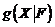by simulation. In the context of option pricing, we regard the X as the variable of logarithmic return rate of the underlying assets, where f is the respective Levy distributional function, andis the respective option’s pricing function. We obtain the sample set by n-time simulations. In this case, we need to evaluate the option’s price on the information set F: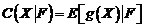Let us consider the sample mean: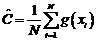(2.1)

What we need is that the sample mean C approximation to the true values, by the law of large numbers can attest, any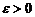, there are: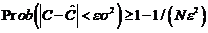(2.2)

The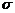is a random variable, the standard deviation of X, the corresponding confidence level can be obtained, under the condition of, the number of simulations N must be determined by the formula: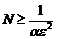(2.3)

Now the confidence interval is within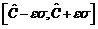. We can infer from this result that with given estimation times, the confidence intervals can be shortened by reducing the variance of random variables to improve the accuracy of estimates. Here note that, with the standard deviation of the random variable being unknown, for some real-world calculation, we can replace it by sample standard deviation.

3. Levy Process Variance Reduction Techniques Under Time-Varying Brown Algorithm

As for the selection of control variable CY, Dingec, Hormann has creatively use the simulation result under the geometric Brownian motion of option Monte Carlo as the control variables with the Levy Process’s generating algorithm. It simplifies the generation of the control variables and facilitates the application to the option pricing. By this means, our study has constructed this variance reduction technique based on the time-varying Brownian algorithm and quasi-Monte Carlo method of Levy process for option pricing. Contrary to the ordinary control variables method for option pricing, this algorithm has the following characteristics:

1.   It introduces the subordinate Brownian motion Levy random number generating algorithm and could be conveniently expanded to most of Levy processes, which makes the variance reduction technique for option pricing more universal.

2.   The control variable CY generated by this technique has a much higher correlation level with the original variable Y. It to a larger part improves the effect of reducing variance.

3.   By quasi Monte Carlo simulation technique, the multi-dimensional random variables are more densely-deployed and the overall computational efficiency is improved. The followings are the details of implementation:

For the underlying asset with the expiration date T, the opening price So and the pricing price, we define the option Y.

The variance reduction algorithm of Levy Monte Carlo on subordinate Brownian motion algorithm has the following steps:

1.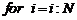where N is the simulation times.

2.     using the Ermontecalo simulation algorithm, generating the time of length T K dimensional uniform distribution of [0,1] number;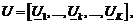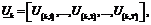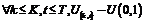3.     We use the first two dimensions of the uniformly-distributed random numbers to generate the one-dimension normal random number B with the length of T by BOX-Muller algorithm.

4.     Using the normal random number B, according to the model and the historical data of option Y, we can generate the random number of return rate subject to geometric Brownian motion. With the remaining normal random numbers, taking B as the basic subordinate Brownian motion, we also generate the return rate series LR by subordinate Brownian motion Levy generating algorithm.

5.     Measure transformation of two groups of random numbers, converted into a risk neutral measure value.

6.     Simulation of path construction of basic assets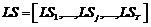,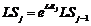by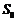and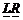; at the same time, Simulation of path construction of basic assets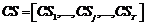,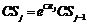byand7.     By Levy process under the asset path, geometric Brown motion path and asset option pricing formula, the first generation of simulated price options: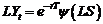and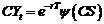8.     Construction of new variables: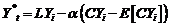9.     End

10. Take the mean of the option price Y by N-time simulation. And take the confidence interval according to the related distributions.

4. The Empirical Results of Variance Reduction

To test the effect of the variance reduction technique of time-varying Brownian motion quasi-Monte Carlo simulation, this section uses European style option S with the underlying asset tied to HSI trading in the Hong Kong Stock Exchange. By adopting NIG, VG as two types of Levy Process and Halton, Sobol as two low-discrepancy sequences, we simulate the option pricing.

NIG process is a random distribution function which is modified out from IG (Inverse Gaussian) process by Barndvrff(1998). Because this type of function has good properties of infinity Diversible, etc, it’s easy to take the model transformation and efficient to generate random numbers. It wide applied to the derivative pricing models under the Levy process(Stentoft,2008 ). VG process is the generalized hyperbolic random function proposed by Madan and Seneta(1990). Since VG process is greatly characterized by the high order moment of financial data and can be generated by two independent Gamma processes, it’s one of the most popular "pure jump-Levy" processes. The selection of low-discrepancy sequences is owing to the high generating efficiency of Halton, simultaneously the evenness of the high-dimensional dispersion.

4.1. The Pricing Results

First, we use the moment estimation method to estimate the logarithm return rate of HSI. Table 1 reports the estimation results. As these parameters are the values under the true measurement, the risk-neutral measure transformation is needed before the derivative pricing.

Table 1. Levy parameter estimation results.

 NIG parameter estimation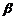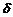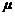9.45E+04 -9.41E+04 0.006784382 0.076036841 FG parameter estimation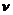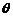0.010353623 0.149199365 -0.008400928 0.004721057

This model has nothing to do with the conditional heteroskedasticity. When measuring the risk neutral level, we can base on the identity function of random process to transform by risk-free return rate. The calculation of the new drift term is: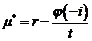(4.1)

Where W is the exponent part of the identity function. respectively.

The identity functions of NIG , VG process are: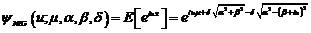(4.2)(4.3)

Based on the previous transformation, we can simulate the logarithm return rate under the risk neutral measurement. Figure 1-2 reports regression results of the simulation distribution and the real-data distribution by NIG and VG models. As the graph shows, the two models for return rate can demonstrate the distribution characteristics of financial data, especially the right-skewed and fat-tailed.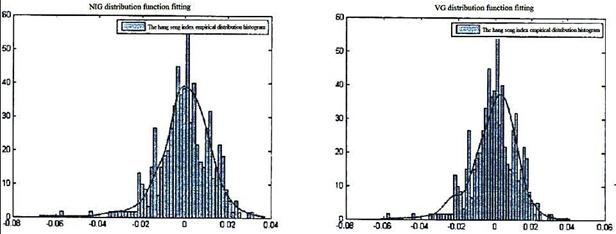Fig. 1. NIG model random distribution fitting.                               Fig. 2. VG model random distribution fitting.

Reduce the data of return rate by simulation to the price path. Them, by the pricing formula of European option, use the "quasi Monte Carlo variance reduction technique" pricing the 25 put and call options with distinct expiration prices respectively.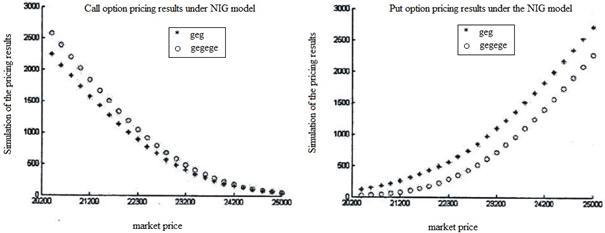Fig. 3. Call option pricing results under the NIG model.                          Fig. 4. Put option pricing results under the NIG model.Fig. 5. Call option pricing results under the VG model.                           Fig. 6. Put option pricing results under the VG model.

In order to compare the NIG, VG models and test the effect of Sobol, Halton series on the pricing result, our study contrasted the pricing methods. Table 2 presents the differences. First, using NIG, VG models, then price the 25 call and put options by Halton and Sobol low-discrepancy sequences respectively. Second, use RMSE (root mean square error) and AAE(average absolute error) to account the pricing result. The two indicators are designed to measure the difference between the pricing result and market price. A smaller error shows a greater precision.

The formulas of these two indicators are: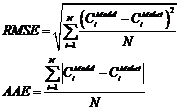(4.4)

Table 2. Levy pricing model results.

 Option type indicators NIG process NIG process VG process VG process Halton sequence Sobol sequence Halton sequence Sobol sequence Call options RMSE 172.6943 182.6824 125.2061 143.389 AAE 158.4619 162.9705 109.6443 121.0722 Put options RMSE 720.1251 579.6429 429.623 455.1074 AAE 708.2064 549.1966 425.8076 431.6199

As is illustrated in the results, the precision is slightly higher than NIG. These two types of Levy process can both help offer a greatly precise pricing. As for the VG process, the pricing results of call options appear to be better than put options. For call options, Halton sequences

4.2. Random Distribution Characteristics

Comparing to ordinary Monte Carlo simulation, the major advantage of "quasi Monte Carlo variance reduction technique" is to accelerate the speed of convergence by applying the quasi Monte Carlo technique and variance reduction technique. Thus, this Section presents the dynamic process of convergence in this simulation by this method.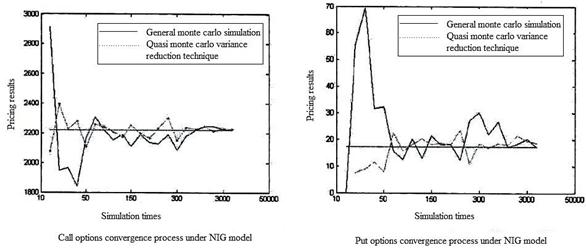Fig. 7. The NIG option pricing process simulation convergence process.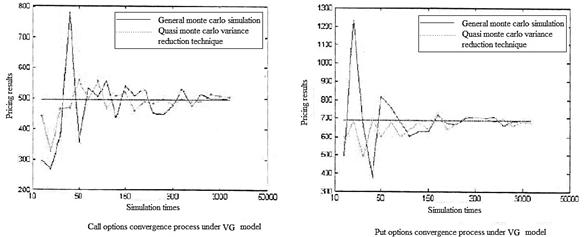Fig. 8. The VG option pricing process simulation convergence process.

Table 2 shows the rates of convergence to the mean with the increase in the simulation times of the pricing results, under the NIG and VC process, respectively by the "quasi Monte Carlo variance reduction technique" and ordinary Monte Carlo simulation. In case of Monte Carlo simulation, the pricing of option requires at least 500 times’ simulation to achieve the convergence to the mean. While taking this variance reduction technique, only 150 times’ simulation can the pricing result be controlled within a reliable range. This shows that, for derivatives’ pricing models, "quasi Monte Carlo variance reduction technique" can effectively reduce the variance and the necessary simulation times, which helps improve the efficiency of pricing.

5. Summary

The application of Levy process can promote the overall pricing performance. At the expense of effectiveness, the Levy process seems more complicated and less efficient than the traditional normal random numbers. Considering ensuring the effectiveness of the pricing result after the use of Monte Carlo simulation technique, a large number of simulations should be taken. Thus how to improve the pricing performance under the circumstance of a low-efficient simulation is critical to all the Levy process Monte Carlo simulation.

In years’ of research, variance reduction techniques are capable of improve the efficiency of Monte Carlo simulation. Moreover, the algorithm is relatively simple. It lays the technical foundation for Levy process variance reduction technique on the time-varying Brownian algorithm in this chapter. In the framework of this algorithm, we combine the following algorithm

1. It corporates the subordinate Brownian motion Levy random number generating algorithm. Because this algorithm is based on the Brownian motion random number, we use the Subordinators to compress and transform the Brownian motion to Levy process. The Brownian motions before and after the compression have a strong association. Thus we use the corresponding Brownian motion as the basis of generating two groups of simulation data. One group is Levy process and the other is the related geometric Brown motion. Then following the framework of control variables method leads to the reduction of variance.

2. The algorithms above involve many a random number. So for the reason of increasing the dispersion evenness, we use the quasi Monte Carlo simulation and match with the Box-Buller algorithm to generate normal random numbers. This offers the basic data support for the Levy process variance reduction techniques on time-varying Brownian algorithm.

Finally, we use warranty dataset in Hong Kong to further the simulation theory. The empirical result shows that this method can help to fasten the rate of convergence of simulation and promote the efficiency of pricing. The precision of pricing can be improved. The specific effects of variance reductions also rest with the styles of options, the type of Levy process and the selection of low-discrepancy sequences.

Acknowledgements

This paper is funded by the project of National Natural Science Fund, Logistics distribution of artificial order picking random process model analysis and research(Project number: 71371033); and funded by intelligent logistics system Beijing Key Laboratory (No.BZ0211); and funded by scientific-research bases--- Science & Technology Innovation Platform---Modern logistics information and control technology research (Project number: PXM2015_014214_000001); and funded by 2014-2015 school year, Beijing Wuzi University, College students' scientific research and entrepreneurial action plan project (No.68); and funded by Beijing Wuzi University, Yunhe scholars program (00610303/007); and funded by Beijing Wuzi University, Management science and engineering Professional group of construction projects. (No. PXM2015_014214_000039). University Cultivation Fund Project of 2014-Research on Congestion Model and algorithm of picking system in distribution center (0541502703)

References

1. Koponen, I. Analytic approach to the problem of convergence of truncated Levy flights towards the Gaussian stochastic process[J]. Physical Review E, 1995,52: 1197-1199.
2. Lays Stentoft. American option pricing using simulation: an introduction with to the GARCH option pricing model[C]. CREATES working paper, 2012.
3. Lehar A, Scheicher M, Schittenkopf C. GARCH vs. stochastic volatility:option pricing and risk management[J]. Journal of Banking&Finance, 2002,160(1): 246-256.
4. Longstaff F A, Schwartz E S. Valuing American options by simulation: a simple least-squares approach[J]. The Review of Financial Studies, 2001, 14(1):113-147.
5. Lydia W. American Monte Carlo option pricing under pure jump Levy models[D]. Stellenbosch University, 2013.
6. Kim J, Jang B G, Kim K T. A simple iterative method for the valuation of American options[J). Quantitative Finance, 2013, 13(6): 885-895.
7. Chorro C, Guegan D, hyperbolic Lelpo F. Option pricing for GARCH-type models with innovation[J] . Finance, 2012, 12(7): 1079-1094.
8. Christoffersen P, Jacobs K, Ornthanalai C. GARCH option valuation:and evidence[Z]. Aarhus University, Working Paper, 2012.theory
9. Byun SJ, Min B. Conditional volatility and the GARCH option pricing model with non-normal innovations[J]. 3ournal of Futures Market, 2413, 33(1): 1-28.
10. Carr P, Madan D B. Option valuation using the fast Fourier transform[J].Journal of Computational Finance, 1999, 2(4): 61-73.
11. Carr P, Geman H, Madan D H and Yor M. The fine structure of asset returns: an empirical investigation[J]. Journal of Business, 2002, 75(2): 305-332.
12. Carr P and Wu L R. The finite moment log stable process and option pricing[J]. Journal of Finance, 2003, 58(2): 753-777.
13. Carriere J F. Valuation of the early exercise price for options using simulations and nonparametric regression[J]. Insurance: Mathematics and Economics, 1996, 19(1): 19-30;

 Contents 1. 2. 3. 4. 4.1. 4.2. 5.
Article ToolsAbstractPDF(1364K)